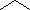×

全部产品
• 全部产品
• 内衣
• 内裤
• 吊带
• 机构
• 钢圈
• 满满一杯
• 软垫
• 软胸罩
• 高顶点
• 跳水
• Dd +杯
• 真丝文胸
• 纯属
• 完整的内裤
• 丁字裤
• 开放

ALL
• ALL
• 32B
• 32C
• 32D
• 32DD
• 32E
• 34B
• 34C
• 34D
• 34DD
• 34E
• 36B
• 36C
• 36D
• 36DD
• 36E
• XS
• S
• M
• L
• XL
• S / M
• M / L
• OS

全部产品
• 全部产品
• Apple
• 黑色
• 蓝色
• 脸红
• 酸果蔓
• 奶油
• 黄金
• 象牙
• 泻湖
• 丁香
• 薄荷
• 裸体
• 粉红色
• 红色
• 阳光
• 白种人
• 黄色